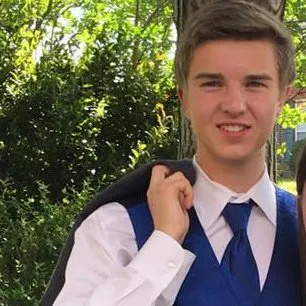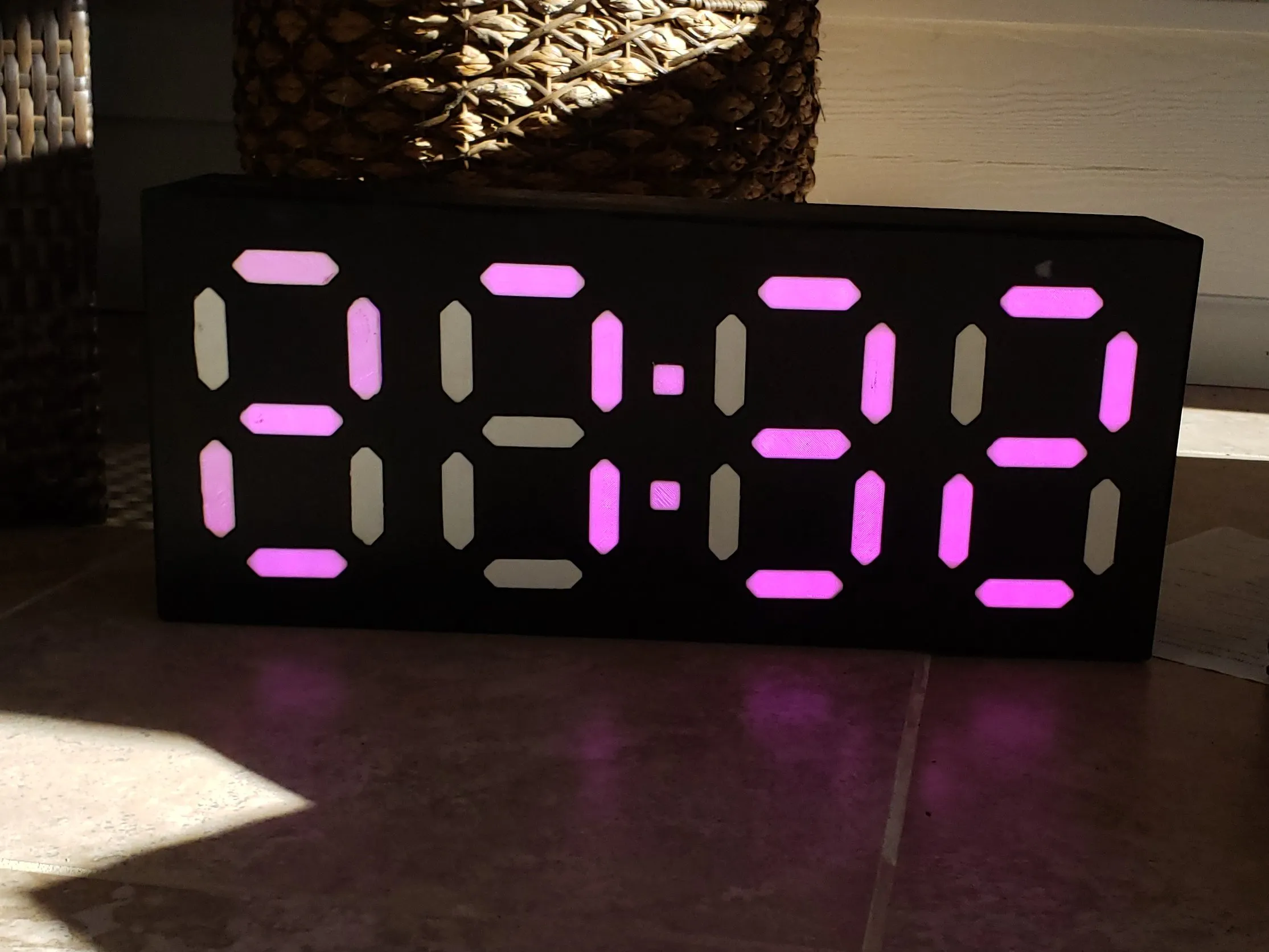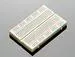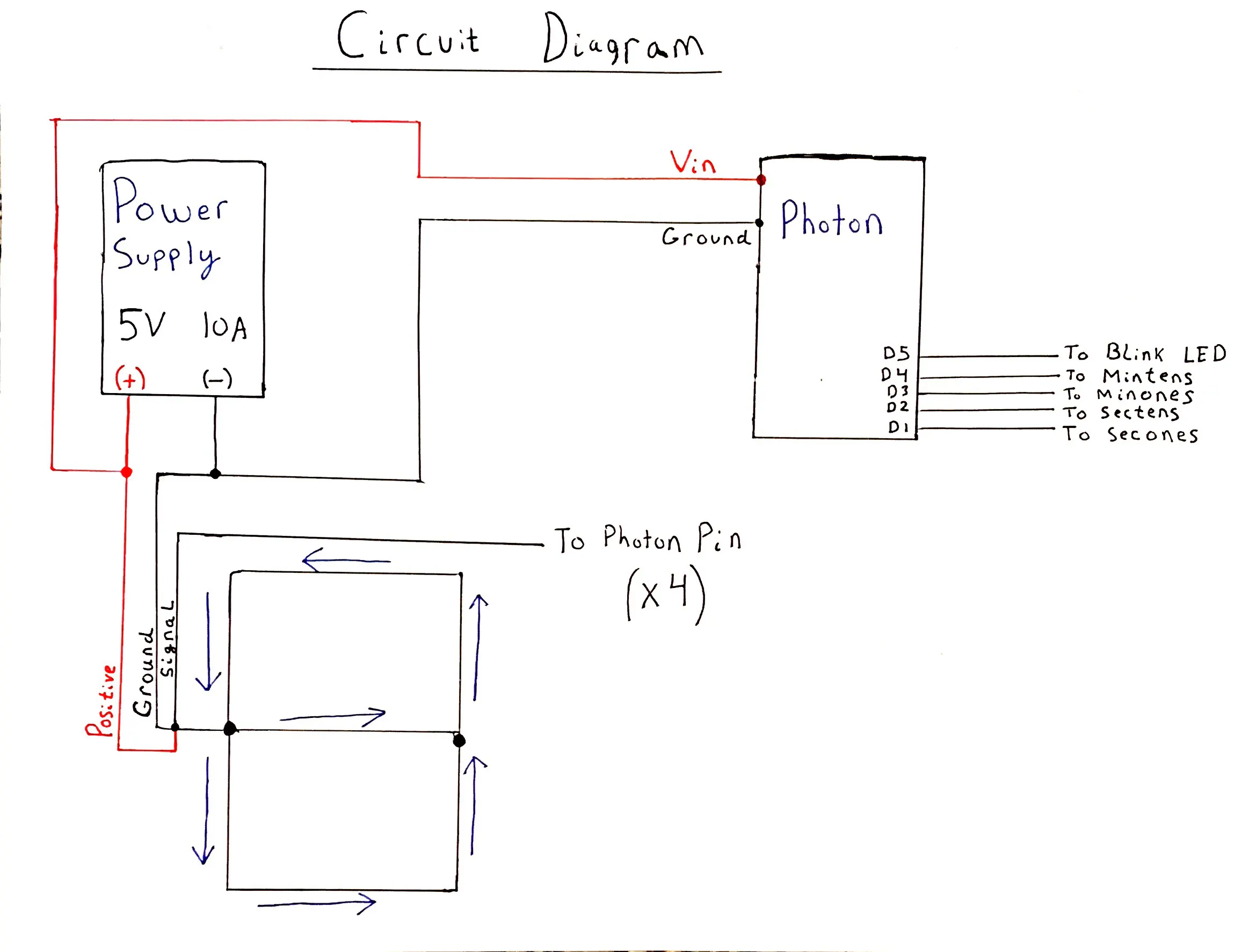Published

# Synced Pace Clocks

IoT Group 17 - A pair of digital pace clocks for the UNC Charlotte Club Swimming Team.

IntermediateShowcase (no instructions)Over 1 day442## Things used in this project

### Hardware components

×1
 5V 10A Power Supply
×2Particle Photon
×2×2
 24x36x1/2" MDF Particle Board
×2

### Software apps and online servicesIFTTT Maker serviceParticle Build Web IDE

### Hand tools and fabrication machines

 Table Saw
 Biscut Joiner
 Soldering Iron
 Router

## Schematics

### Clock Schematic## Code

### Master Clock

C/C++
```#include <neopixel.h>

#include "Particle.h"

#define PIXEL_COUNT 21
#define PIXEL_TYPE WS2812B

int r = 255;
int g = 0;
int b = 0;

};

int i = 0;

void myHandler(const char *event, const char *data)
{
i++;
Serial.print(i);
Serial.print(event);
Serial.print(", data: ");
if (data)
Serial.println(data);
else
Serial.println("not connected");
}

void setup()

{
Particle.publish("lights0227", "clocks connected");

Particle.subscribe("lights0228", myHandler);

for(int s=0;s<5;s++)
{
pixels[s].begin();
pixels[s].show(); // Initialize all pixels to 'off'
}

}

void loop(){

int secs = Time.second();
int mins = Time.minute();

int secsTens = secs/10; //get the tens place of seconds
int secsOnes = secs%10; //get the ones place of seconds
int minsTens = mins/10; //get the tens place of minutes
int minsOnes = mins%10; //get the ones place of minutes

drawdigit(0, 0, r, g, b, secsOnes);
pixels.show();

drawdigit(1, 0, r, g, b, secsTens);
pixels.show();

pixels.setPixelColor(0,  r, g, b);
pixels.setPixelColor(1,  r, g, b);
pixels.show();

}

void drawdigit(int s, int offset, int r, int g, int b, int n){
if (n==2 || n==3 || n== 4 || n==5 || n==6 || n==8 || n==9 )//MIDDLE
{
pixels[s].setPixelColor(0, pixels[s].Color(r,g,b));
pixels[s].setPixelColor(1, pixels[s].Color(r,g,b));
pixels[s].setPixelColor(2, pixels[s].Color(r,g,b));
}
else
{
pixels[s].setPixelColor(0, pixels[s].Color(0,0,0));
pixels[s].setPixelColor(1, pixels[s].Color(0,0,0));
pixels[s].setPixelColor(2, pixels[s].Color(0,0,0));
}
if (n==0 || n==1 || n== 2 || n==3 || n==4 || n==7 || n==8 || n==9)//TOP RIGHT
{
pixels[s].setPixelColor(3, pixels[s].Color(r,g,b));
pixels[s].setPixelColor(4, pixels[s].Color(r,g,b));
pixels[s].setPixelColor(5, pixels[s].Color(r,g,b));
}
else
{
pixels[s].setPixelColor(3, pixels[s].Color(0,0,0));
pixels[s].setPixelColor(4, pixels[s].Color(0,0,0));
pixels[s].setPixelColor(5, pixels[s].Color(0,0,0));
}
if (n==0 || n==2 || n== 3 || n==5 || n==6 || n==7 || n==8 || n==9)//TOP
{
pixels[s].setPixelColor(6, pixels[s].Color(r,g,b));
pixels[s].setPixelColor(7, pixels[s].Color(r,g,b));
pixels[s].setPixelColor(8, pixels[s].Color(r,g,b));
}
else
{
pixels[s].setPixelColor(6, pixels[s].Color(0,0,0));
pixels[s].setPixelColor(7, pixels[s].Color(0,0,0));
pixels[s].setPixelColor(8, pixels[s].Color(0,0,0));
}
if (n==0 || n==4 || n==5 || n== 6 || n==8 || n==9 )//TOP LEFT
{
pixels[s].setPixelColor(9, pixels[s].Color(r,g,b));
pixels[s].setPixelColor(10, pixels[s].Color(r,g,b));
pixels[s].setPixelColor(11, pixels[s].Color(r,g,b));
}
else
{
pixels[s].setPixelColor(9, pixels[s].Color(0,0,0));
pixels[s].setPixelColor(10, pixels[s].Color(0,0,0));
pixels[s].setPixelColor(11, pixels[s].Color(0,0,0));
}
if (n==0 || n==2 || n==6 || n== 8)//BOTTOM LEFT
{
pixels[s].setPixelColor(12, pixels[s].Color(r,g,b));
pixels[s].setPixelColor(13, pixels[s].Color(r,g,b));
pixels[s].setPixelColor(14, pixels[s].Color(r,g,b));
}
else
{
pixels[s].setPixelColor(12, pixels[s].Color(0,0,0));
pixels[s].setPixelColor(13, pixels[s].Color(0,0,0));
pixels[s].setPixelColor(14, pixels[s].Color(0,0,0));
}
if (n==0 || n==2 || n==3 || n== 5 || n==6 || n==8 || n==9)//BOTTOM
{
pixels[s].setPixelColor(15, pixels[s].Color(r,g,b));
pixels[s].setPixelColor(16, pixels[s].Color(r,g,b));
pixels[s].setPixelColor(17, pixels[s].Color(r,g,b));
}
else
{
pixels[s].setPixelColor(15, pixels[s].Color(0,0,0));
pixels[s].setPixelColor(16, pixels[s].Color(0,0,0));
pixels[s].setPixelColor(17, pixels[s].Color(0,0,0));
}
if (n==0 || n==1 || n==3 || n==4 || n==5 || n==6|| n==7 || n==8 || n==9)//BOTTOM RIGHT
{
pixels[s].setPixelColor(18, pixels[s].Color(r,g,b));
pixels[s].setPixelColor(19, pixels[s].Color(r,g,b));
pixels[s].setPixelColor(20, pixels[s].Color(r,g,b));
}
else
{
pixels[s].setPixelColor(18, pixels[s].Color(0,0,0));
pixels[s].setPixelColor(19, pixels[s].Color(0,0,0));
pixels[s].setPixelColor(20, pixels[s].Color(0,0,0));
}
pixels[s].show();
}
```

### Slave Clock

C/C++
```#include <neopixel.h>

// This #include statement was automatically added by the Particle IDE.

#include "Particle.h"

#define PIXEL_COUNT 21
#define PIXEL_TYPE WS2812B

int r = 255;
int g = 0;
int b = 0;

};

int i = 0;

void myHandler(const char *event, const char *data)
{
i++;
Serial.print(i);
Serial.print(event);
Serial.print(", data: ");
if (data)
Serial.println(data);
else
Serial.println("not connected");
}

void setup()

{
Particle.subscribe("lights0227", myHandler);

Particle.publish("lights0228", "clocks connected");

for(int s=0;s<5;s++)
{
pixels[s].begin();
pixels[s].show(); // Initialize all pixels to 'off'
}

}

void loop(){

int secs = Time.second();
int mins = Time.minute();

int secsTens = secs/10; //get the tens place of seconds
int secsOnes = secs%10; //get the ones place of seconds
int minsTens = mins/10; //get the tens place of minutes
int minsOnes = mins%10; //get the ones place of minutes

drawdigit(0, 0, r, g, b, secsOnes);
pixels.show();

drawdigit(1, 0, r, g, b, secsTens);
pixels.show();

drawdigit(2, 0, r, g, b, minsOnes);
pixels.show();

drawdigit(3, 0, r, g, b, minsTens);
pixels.show();

pixels.setPixelColor(0,  r, g, b);
pixels.setPixelColor(1,  r, g, b);
pixels.show();

}

void drawdigit(int s, int offset, int r, int g, int b, int n){
if (n==2 || n==3 || n== 4 || n==5 || n==6 || n==8 || n==9 )//MIDDLE
{
pixels[s].setPixelColor(0, pixels[s].Color(r,g,b));
pixels[s].setPixelColor(1, pixels[s].Color(r,g,b));
pixels[s].setPixelColor(2, pixels[s].Color(r,g,b));
}
else
{
pixels[s].setPixelColor(0, pixels[s].Color(0,0,0));
pixels[s].setPixelColor(1, pixels[s].Color(0,0,0));
pixels[s].setPixelColor(2, pixels[s].Color(0,0,0));
}
if (n==0 || n==1 || n== 2 || n==3 || n==4 || n==7 || n==8 || n==9)//TOP RIGHT
{
pixels[s].setPixelColor(3, pixels[s].Color(r,g,b));
pixels[s].setPixelColor(4, pixels[s].Color(r,g,b));
pixels[s].setPixelColor(5, pixels[s].Color(r,g,b));
}
else
{
pixels[s].setPixelColor(3, pixels[s].Color(0,0,0));
pixels[s].setPixelColor(4, pixels[s].Color(0,0,0));
pixels[s].setPixelColor(5, pixels[s].Color(0,0,0));
}
if (n==0 || n==2 || n== 3 || n==5 || n==6 || n==7 || n==8 || n==9)//TOP
{
pixels[s].setPixelColor(6, pixels[s].Color(r,g,b));
pixels[s].setPixelColor(7, pixels[s].Color(r,g,b));
pixels[s].setPixelColor(8, pixels[s].Color(r,g,b));
}
else
{
pixels[s].setPixelColor(6, pixels[s].Color(0,0,0));
pixels[s].setPixelColor(7, pixels[s].Color(0,0,0));
pixels[s].setPixelColor(8, pixels[s].Color(0,0,0));
}
if (n==0 || n==4 || n==5 || n== 6 || n==8 || n==9 )//TOP LEFT
{
pixels[s].setPixelColor(9, pixels[s].Color(r,g,b));
pixels[s].setPixelColor(10, pixels[s].Color(r,g,b));
pixels[s].setPixelColor(11, pixels[s].Color(r,g,b));
}
else
{
pixels[s].setPixelColor(9, pixels[s].Color(0,0,0));
pixels[s].setPixelColor(10, pixels[s].Color(0,0,0));
pixels[s].setPixelColor(11, pixels[s].Color(0,0,0));
}
if (n==0 || n==2 || n==6 || n== 8)//BOTTOM LEFT
{
pixels[s].setPixelColor(12, pixels[s].Color(r,g,b));
pixels[s].setPixelColor(13, pixels[s].Color(r,g,b));
pixels[s].setPixelColor(14, pixels[s].Color(r,g,b));
}
else
{
pixels[s].setPixelColor(12, pixels[s].Color(0,0,0));
pixels[s].setPixelColor(13, pixels[s].Color(0,0,0));
pixels[s].setPixelColor(14, pixels[s].Color(0,0,0));
}
if (n==0 || n==2 || n==3 || n== 5 || n==6 || n==8 || n==9)//BOTTOM
{
pixels[s].setPixelColor(15, pixels[s].Color(r,g,b));
pixels[s].setPixelColor(16, pixels[s].Color(r,g,b));
pixels[s].setPixelColor(17, pixels[s].Color(r,g,b));
}
else
{
pixels[s].setPixelColor(15, pixels[s].Color(0,0,0));
pixels[s].setPixelColor(16, pixels[s].Color(0,0,0));
pixels[s].setPixelColor(17, pixels[s].Color(0,0,0));
}
if (n==0 || n==1 || n==3 || n==4 || n==5 || n==6|| n==7 || n==8 || n==9)//BOTTOM RIGHT
{
pixels[s].setPixelColor(18, pixels[s].Color(r,g,b));
pixels[s].setPixelColor(19, pixels[s].Color(r,g,b));
pixels[s].setPixelColor(20, pixels[s].Color(r,g,b));
}
else
{
pixels[s].setPixelColor(18, pixels[s].Color(0,0,0));
pixels[s].setPixelColor(19, pixels[s].Color(0,0,0));
pixels[s].setPixelColor(20, pixels[s].Color(0,0,0));
}
pixels[s].show();
}
```

## Credits

### Colin Catella

1 project • 0 followers